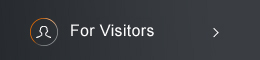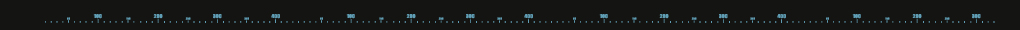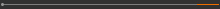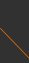Sponsored by[ Events ]

﻿
Activity Search
Sort out
 select all Seminars and Talks Conference and Workshop Lectures and Courses ElseField
 select all Number Theory and Representation Theory Algebraic Geometry Differential Geometry and Geometric Analysis Differential Equations and Stochastic Analysis Scientific Computing Interdisciplinary Studies Laboratory of Data Science Harmonic Analysis OthersYear
 select 2015 2016 2017 2018 2019 2020 2021 20222021 NCTS Seminar on Dynamical Systems16:00 - 17:00, October 26, 2021 (Tuesday) Zoom, Online seminar (線上演講 Zoom) The Entropy of Multiplicative Subshifts on Trees Zongfan Zhang (Sichuan University) Abstract: In this talk, we will discuss the multiplicative integer systems on trees, those are countable graphs with no loop, and compute their entropies, which are usually used to measure the complexity of them. This talk is divided into two parts. In part I, we first introduce the q-multiplicative subshift on trees (q-MST) and present an explicit formula for the entropy of q-MST. Also, the coupled q-MST is considered, which is the intersection of a q-MST and a tree subshift. In a particular class, we provide a lower bound of the entropy of the coupled q-MST by using the entropy of its associated tree subshift. In part II, we study the multiple multiplicative subshift on trees (MMST). When the subshift is a one-step subshift of finite type, the explicit entropy formula can be obtained under certain conditions. Join Zoom Meeting(C) 2021 National Center for Theoretical Sciences